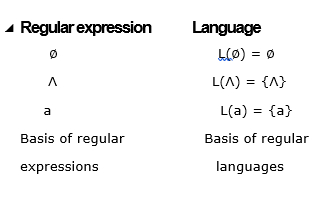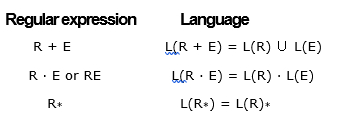# Explain about regular expressions in TOC?

A regular expression is basically a shorthand way of showing how a regular language is built from the base set of regular languages.

The symbols are identical which are used to construct the languages, and any given expression that has a language closely associated with it.

For each regular expression E, there is a regular language L(E).

Example 1

If the regular expression is as follows −

a + b · a*

It can be written in fully parenthesized form as follows −

(a + (b · (a*)))

Regular expressions vs. Languages

The symbols of the regular expressions are distinct from those of the languages. These symbols are given below −Operators in Regular expression −

There are two binary operations on regular expressions (+ and ·) and one unary operator (*)These are closely associated with the union, product and closure operations on the corresponding languages.

Example 1

The regular expression a + bc* is basically shorthand for the regular language {a} ∪ ({b} · ({c}*)).

Example 2

Find the language of the given regular expression. It is explained below −

a + bc*.
L(a + bc*) = L(a) ∪ L(bc*)
= L(a) ∪ (L(b) · L(c*))
= L(a) ∪ (L(b) · L(c)*)
= {a} ∪ ({b} · {c}*)
= {a} ∪ ({b} · {∧, c, c2, . . . , cn, . . . , })
= {a} ∪ {b, bc, bc2, . . . , bcn, . . . }
= {a, b, bc, bc2, . . . , bcn, . . . }.